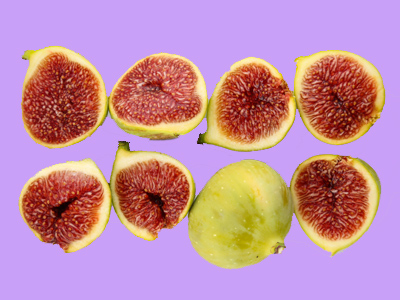8 can be divided into 2 groups of 4 or 4 groups of 2.

# Year 1 Calculation - Division and Sharing

This quiz addresses the requirements of the National Curriculum KS1 Maths and Numeracy for children aged 5 and 6 in year 1. Specifically this quiz is aimed at the section dealing with division using concrete objects, pictorial representations and arrays.

Division in Year 1 usually means sharing into equal groups. This can be related to repeated subtraction and pictures, representations, arrays or objects. These are often used to support this. Division facts can be related to the correct multiplication. For example: if a child knows that 2 x 4 = 8,
then they should recognise that 8 ÷ 4 = 2, or 8 ÷ 2 = 4

This quiz will help children to become familiar with divisions using representations and arrays.

Question 1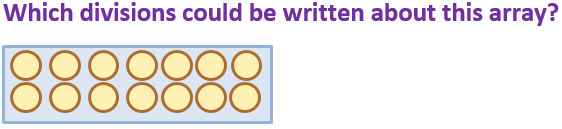16 ÷ 8 = 2 and 16 ÷ 2 = 8
10 ÷ 5 = 2 and 10 ÷ 2 = 5
12 ÷ 2 = 6 and 12 ÷ 6 = 2
14 ÷ 7 = 2 and 14 ÷ 2 = 7
There are 14 circles altogether
Question 2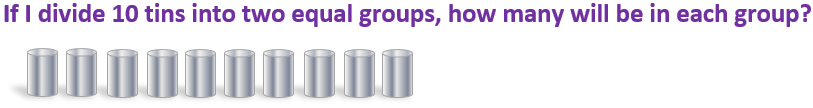8
11
10
5
10 ÷ 2 = 5
Question 3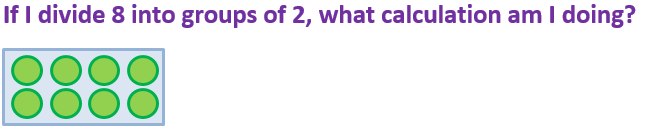8 ÷ 2
2 ÷ 8
16 ÷ 8
12 ÷ 2
Sharing into groups of 2 is the same as dividing by 2
Question 4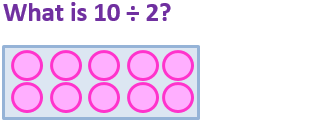10
5
2
20
10 ÷ 2 means sharing 10 into 2 equal groups, with 5 in each group
Question 5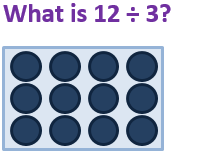30
12
8
4
12 shared into 3 equal groups gives 4 in each group
Question 6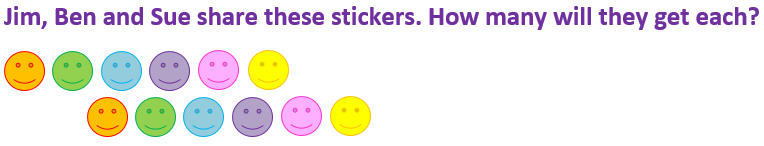12 x 2 = 24
12 ÷ 4 = 3
12 x 3 = 36
12 ÷ 3 = 4
There are 12 stickers to be shared between 3 people, so 12 ÷ 3 = 4
Question 7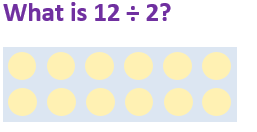22
16
6
12
12 shared into 2 equal groups is 6
Question 8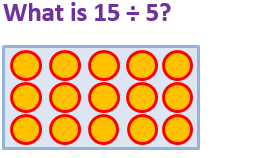35
5
15
3
15 shared into 5 equal groups gives 3 in each group
Question 9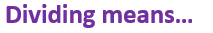Finding the total
Sharing into equal groups
Making numbers bigger
Dividing is the same as sharing into groups
Question 10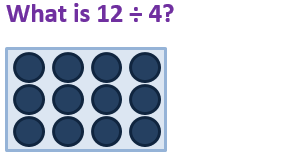3
4
12
8
12 ÷ 4 = 3 and 12 ÷ 3 = 4